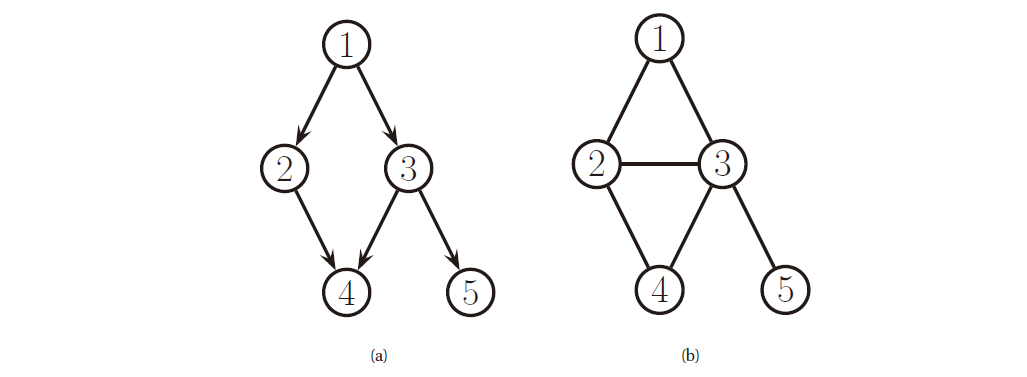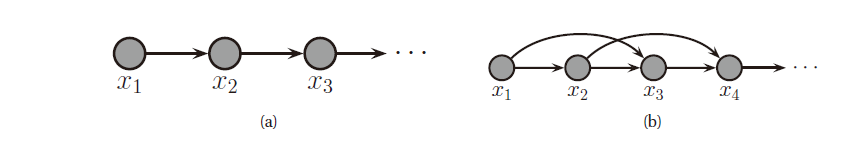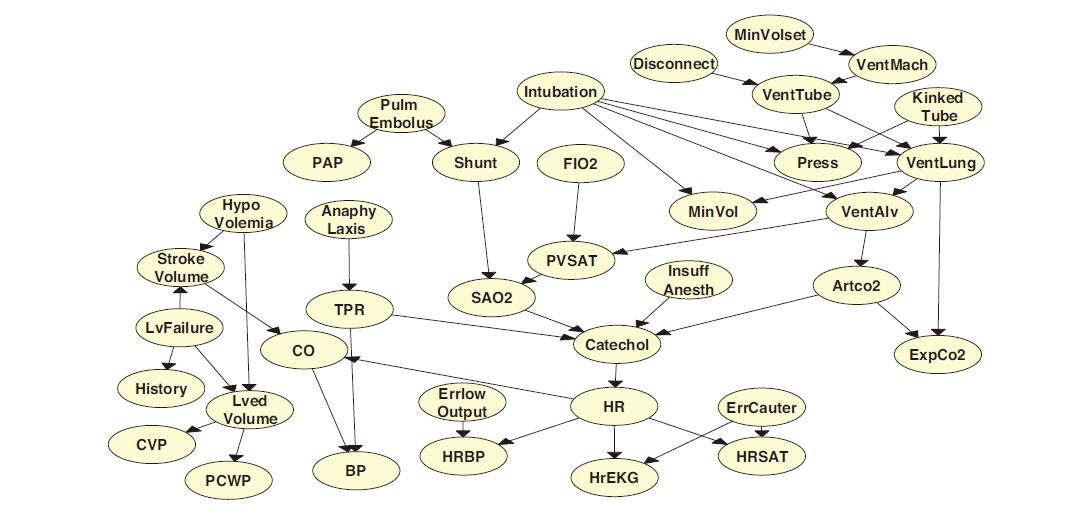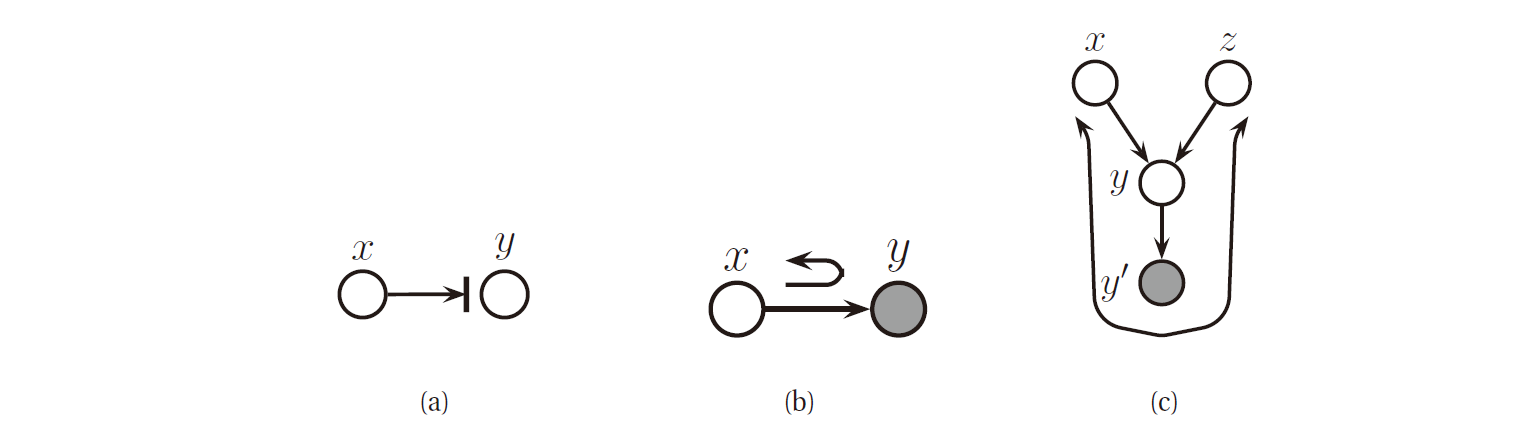Jarvis' Blog 总有美丽的风景让人流连

# 机器学习(三): 有向图模型 (Directed Graphical Models)

2020-08-19
Jarvis
Post

1. 模块化原理
2. 抽象化原理

– Michael Jordan

$\newcommand{\x}{\mathbf{x}} \newcommand{\tb}{\boldsymbol{\theta}}$ 假设我们观测到了多个互相关联的变量, 如文档中的单词, 图像中的像素, 或者基因. 那么我们如何紧凑的表示多个关联变量的联合分布 $p(\x\vert\tb)$ 呢? 我们如何使用这个分布在给定一些变量之后推理另一些变量呢?

## 1. 介绍

### 1.1 链式法则

$p(\x_{1:V})=p(x_1)p(x_2\vert x_1)p(x_3\vert x_2,x_1)p(x_4\vert x_1,x_2,x_3)\dots p(x_V\vert x_{1:V-1})$

### 1.2 条件独立

$X\perp Y\vert Z \Longleftrightarrow p(X,Y\vert Z)=p(X\vert Z)p(Y\vert Z)$

$p(\x_{1:V})=p(x_1)\prod_{t=1}^Vp(x_t\vert x_{t-1})$

### 1.3 图模型### 1.4 有向图模型

$x_s\perp\x_{pred(s)\backslash pa(s)}\vert \x_{pa(s)}$

\newcommand{\cancel}{\enclose{updiagonalstrike}} \begin{align}\require{enclose} p(\x_{1:5}) &= p(x_1)p(x_2\vert x_1)p(x_3\vert x_1,\cancel{x_2})p(x_4\vert \cancel{x_1},x_2,x_3)p(x_5\vert \cancel{x_1}\cancel{x_2},x_3,\cancel{x_4}) \\ &= p(x_1)p(x_2\vert x_1)p(x_3\vert x_1)p(x_4\vert x_2,x_3)p(x_5\vert x_3) \end{align}

$p(\x_{1:V}\vert G)=\prod_{t=1}^Vp(x_t\vert \x_{pa(t)})$

## 2. 例子

### 2.1 朴素贝叶斯分类器$p(y,\x)=p(y)\prod_{j=1}^Dp(x_j\vert y)$

### 2.2 马尔可夫和隐马尔可夫模型### 2.3 医疗诊断

• ICU 中的报警网络 (alarm network)• 诊断时的快速医疗参考 (quick medical reference)网络## 3. 推断

$p(\x_h\vert \x_v,\tb)=\frac{p(\x_h,\x_v\vert \tb)}{p(\x_v\vert \tb)}=\frac{p(\x_h,\x_v\vert \tb)}{\sum_{\x_h'}p(\x_h',\x_v\vert \tb)}$

$p(\x_q\vert \x_v,\tb)=\sum_{\x_n}p(\x_q,\x_n\vert \x_v,\tb)$

## 4. 学习

$\hat{\tb}=\underset{\tb}{\arg\max}\sum_{i=1}^N\log p(\x_{i,v}\vert \tb)+\log p(\tb)$

## 5. DGMs 中的条件独立性

### 5.1 d-划分和贝叶斯球算法 (全局马尔可夫性)

1. $P$ 包含一条链, $s\rightarrow m\rightarrow t$ 或 $s\leftarrow m\leftarrow t$ , 其中 $m\in E$
2. $P$ 包含一个分叉, $s\swarrow^m\searrow t$ , 其中 $m\in E$
3. $P$ 包含一个 v 型结构, $s\searrow_m\swarrow t$ , 其中 $m\notin E$ , 并且 $m$ 的任何后代也不属于 $E$ .

$\x_A\perp_G\x_B\vert \x_E \Longleftrightarrow A \text{ is d-separated from } B \text{ given } E.$1. 小球可以通过一个链, 但中间存在观测变量时无法通过
2. 小球可以通过分叉结构, 但中间存在观测变量时无法通过
3. 小球可以通过 V-型结构, 但中间存在观测变量时可以通过

#### 5.1.1 链

$p(x,y,z)=p(x)p(y\vert x)p(z\vert y)$

$p(x,z\vert y)=\frac{p(x)p(y\vert x)p(z\vert y)}{p(y)}=\frac{p(x,y)p(z\vert y)}{p(y)}=p(x\vert y)p(z\vert y)$

#### 5.1.2 分叉

$p(x,y,z)=p(y)p(x\vert y)p(z\vert y)$

$p(x,z\vert y)=\frac{p(y)p(x\vert y)p(z\vert y)}{p(y)}=p(x\vert y)p(z\vert y)$

#### 5.1.3 V-型结构

$p(x,y,z)=p(x)p(z)p(y\vert x,z)$

$p(x,z\vert y)=\frac{p(x)p(z)p(x,z\vert y)}{p(y)}$

$p(x,z)=p(x)p(z)$

#### 5.1.4 边界条件• $2\rightarrow5\rightarrow6$ 被 $x_5$ 阻断 ( $x_5$ 被观测到了)
• $2\rightarrow4\rightarrow7\rightarrow6$ 被 $x_7$ 阻断 ( $x_7$ 没有被观测到)
• $2\rightarrow1\rightarrow3\rightarrow6$ 被 $x_1$ 阻断 ( $x_1$ 被观测到了)

### 5.2 DGMs 的其他马尔可夫性质

#### 5.2.1 有向局部马尔可夫性 L

$t\perp nd(t)\backslash pa(t)\vert pa(t)$

#### 5.2.2 有序马尔可夫性 O

$t\perp pred(t)\backslash pa(t)\vert pa(t)$

#### 5.2.3 有向全局马尔可夫性 G

$\x_A\perp_G\x_B\vert \x_E \Longleftrightarrow A \text{ is d-separated from } B \text{ given } E.$

### 5.3 马尔可夫覆盖和全条件

$mb(t)\triangleq ch(t)\cup pa(t)\cup copa(t)$

$mb(5)=\{6,7\}\cup\{2\}\cup\{4\}=\{2,4,6,7\}$

$p(x_t\vert\x_{-t})\propto p(x_t\vert\x_{pa(t)})\prod_{s\in ch(t)}p(x_s\vert\x_{pa(s)})$

## 参考文献

1. Machine Learning: A Probabilistic Perspective
Kevin P. Murphy
[html], [pdf], [index], Chapter 10, Directed graphical models (Bayes nets)  2

2. MAJOR bug fixes/changes to appear in printing #4 (out September 2013)
[html] [index]  2

3. Directed Graphical Models
John Lafferty, Han Liu, and Larry Wasserman
[pdf], Chapter 18

Content